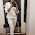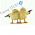# Differentiate between standardization and specification giving appropriate examples. How are 'preferred numbers' useful in standardization? Explain with the help of a suitable example.

Standardization is the process of establishing standards or units of measure by which extent, quality, quantity, value performance, may be compared and measured.

The following standards are used for designing products:
(i) Standards for materials, their chemical compositions, their physical properties (e.g. mechanical) and heat treatment.
(ii) Standards for fits, tolerances and surface finish of components.
(iii) Different shapes and dimensions of commonly used machine elements e.g., nuts, bolts, rivets, gears etc.

Now the product specification includes such as dimensions of the components e.g. Volume, area, weight, speed, load, power etc. are expressed numerically e.g. 10 kw, 20 kN, 500 r.p.m or 10 kg etc.

Preferred numbers are used to specify such specification because a company may manufacture different models of same product.

The system is based on the use of geometric progressive to develop a set of numbers.

There are five basic series, denoted by R5, R10, R20, R40 and R80, which increased in steps of 58%, 26%, 12%, 6% and 3% respectively.

The series is obtained by taking the first number and multiplying it by a series factor to get the second number.

E.g. Consider a company manufacture of lifting machine who wants to introduce six different models of capacities ranging from 50kN to 100kN.

Considering R20 series, the capacity of different models of lifting machine will be 55 KN using R20 series--> 20/10 = 1.12

55 * 1.12 = 61.6 = 61 KN,
61 * 1.12 = 68.32 = 68kN,
68 * 1.12 = 76.16 = 70kN,
76 * 1.12 = 85.12 = 85kN,
85 * 1.12 = 95.2 = 95kN

1.first of all in the third last line, I think it should be 76kN.
secondly "Considering R20 series, the capacity of different models of lifting machine will be 55 KN using R20 series--> 20/10 = 1.12" would you please elaborate this line.

2.1.3.That is an extremely smart written article. I will be sure to bookmark it and This article is efficient. Thank you for sharing it with us. I am visiting this blog on a daily basis and I am finding so much helpful article each time. Keep working on this and thank you once again...

www.mixresult.com, a new-generation Mix education result news portal, publishes update result news from a highly interactive platform.

www.mixresult.com